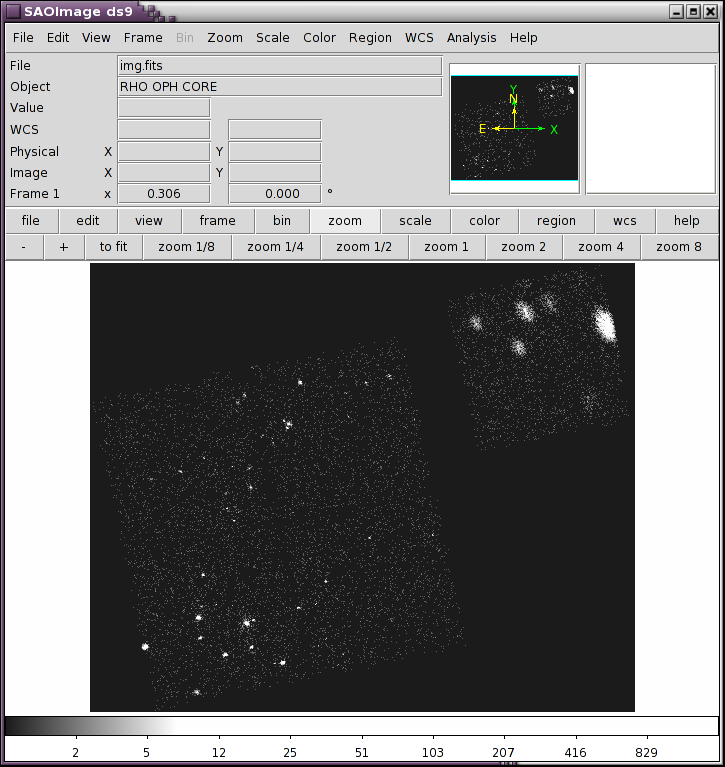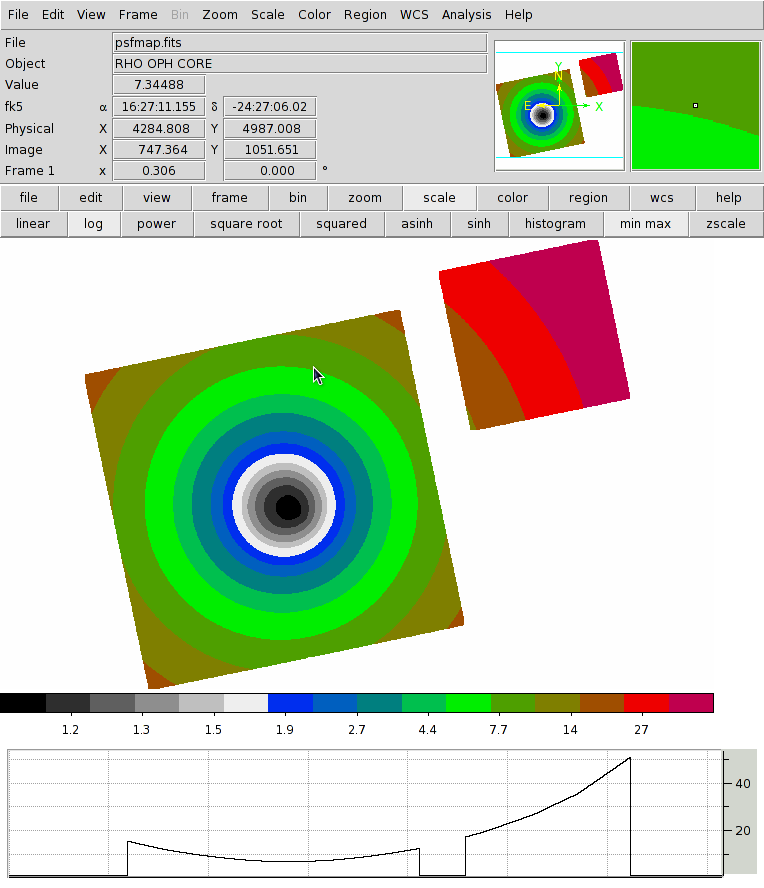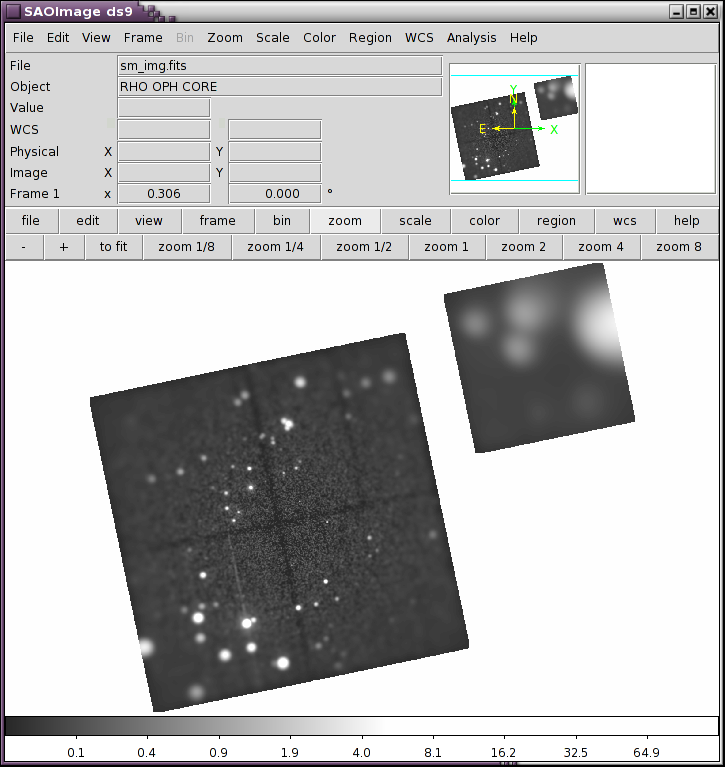# PSF Size Image Smoothing## Overview

#### Synopsis:

The Chandra Point Spread Function varies considerably across the field of view, from nearly a single pixel on-axis to several hundred pixels at off-axis angles > 10'. It can be tricky to smooth the image such that each pixel is smoothed by something that represents the same intrinsic PSF size at each point.

In this thread we show one technique that allows an image to be smoothed such that each pixel is smoothed at the same scale as the PSF.

#### Purpose:

This thread shows how to smooth an image such that each pixel is smoothed at the size of the PSF.

## Getting Started

`unix% download_chandra_obsid 635 evt2,fov`

To begin we will bin the event file into an image. Here we filter the event list on energy in the 0.5 to 7.0 keV range, and apply a region filter in the form of the field of view file. The FOV filter allows the tool to know the active area of the image space and when it is then binned, the image, Figure 1, is made such that it just encloses the FOV rather than the full 8k x 8k image space.

```unix% dmcopy "acisf00635N004_evt2.fits.gz[energy=500:7000,sky=region(acisf00635_000N004_fov1.fits.gz)][bin sky=2]" img.fits clob+
unix% dmlist img.fits cols

--------------------------------------------------------------------------------
Columns for Image Block EVENTS_IMAGE
--------------------------------------------------------------------------------

ColNo  Name                 Unit        Type             Range
1   EVENTS_IMAGE[1780,1469]              Int2(1780x1469) -

--------------------------------------------------------------------------------
Physical Axis Transforms for Image Block EVENTS_IMAGE
--------------------------------------------------------------------------------

Group# Axis#
1   1,2    sky(x) = (+2791.0795) +(+2.0)* ((#1)-(+0.50))
(y)   (+2884.7055)  (+2.0)  ((#2) (+0.50))

--------------------------------------------------------------------------------
World Coordinate Axis Transforms for Image Block EVENTS_IMAGE
--------------------------------------------------------------------------------

Group# Axis#
1   1,2    EQPOS(RA ) = (+246.8247)[deg] +TAN[(-0.000136667)* (sky(x)-(+4096.50))]
(DEC)   (-24.5734 )           (+0.000136667)  (   (y) (+4096.50))
```

### Figure 1: Image of Rho Oph Field### Figure 1: Image of Rho Oph Field

Image of the Rho Oph field in the 0.5 to 7.0 keV range, binned by 2.

## Determine the Size of the PSF

To smooth at the same size as the PSF, first we need to know what that is. The mkpsfmap tool can be used to do just this. We do however need to decide on two parameters. What energy? What PSF fraction?

The size of the PSF varies with energy, though less than it does by off-axis-angle. We should choose an energy that is appropriate for the energy of the events in the image. For this example we choose an energy of 2.3keV which is the same energy chosen by the Chandra Source Catalog for this energy band ("broad").

The second question will depend on your specific task. What fraction of the PSF are you expecting to include? To include 100% of the PSF would require an infinite smoothing radius. For this exercise we choose a PSF size of 90%; that is it is expected that 90% of the PSF would fall within a circle with this radius.

```unix% mkpsfmap infile=img.fits outfile=psfmap.fits energy=2.3 ecf=0.9 units=logical
```

It is important here to note that we chose units=logical. This tells mkpsfmap to compute the size of the PSF in unit of pixel as the image is currently binned (in this example by 2).

The output image looks like Figure 2

### Figure 2: A map of the PSF size### Figure 2: A map of the PSF size

A map of the 2.3 keV, 90% PSF size for obsid 635. Values are scaled logarithmically and uses the "color" color map. The bottom plot shows a horizontal slice through the data showing radial dependence. Also note that values outside the image are set to NaN. This is because the input image had the FOV region applied, and so the tool knew which pixels were off the detector and thus have no PSF size.

## Truncate Values

For the next step we require a reasonably finite number of smoothing scales. The output of mkpsfmap though is continuous and if used as is, it would exhaust all system resources. Therefore before we proceed we will truncate the values. We can truncate at any reasonable precision so we will choose to truncate at the 1st decimal point using dmimgcalc. We also apply a threshold, any values that are less than 0.1 pixels are reset to be 0.1. This ensure that all pixels will have some smoothing applied.

```unix% dmimgcalc psfmap.fits none trunk_psf.fits op="imgout=((int)(img1*10.0))/10.0"
unix% dmimgthresh trunk_psf.fits tt_psf.fits cut=0.1 value=0.1
```

By eye, the image looks very similar to Figure 2, with the exception that values outside the FOV are now set to 0.1 instead of being NaN.

We are now ready to smooth the original image. For this exercise we will smooth with a cone whose base radius is equal to the PSF size. To do this we use the dmimgadapt tool.

```unix% dmimgadapt infile=img.fits outfile=sm_img.fits function=cone \
Pre-computing convolution kernels
First iteration: determine scales and normalization
Percent complete: 99.93%
Second iteration: computing final normalized values```

With this size image and the number of scales, this will take a few minutes to run. We could also use a gaussian instead of a cone but it will take roughly 15min (depending on hardware) since the Gaussian has greater extent. With verbose=3 the percent complete is updated with each row. The output is shown in Figure 3.

### Figure 3: Image smoothed at size of PSF### Figure 3: Image smoothed at size of PSF

Each pixel has been smoothed at the size of the PSF and renormalized based on the neighboring smoothing scales to preserve flux. Data near the optical axis have been barely smoothed while away from the optical axis the image has been heavily smoothed.

The output image also has NaNs for the data points outside the original FOV filter.

## Summary

This kind of image can be used to diagnose whether structure seen in an off-axis source is instrumental (PSF) or astrophysical. Since convolution and correlation are related, it can also be used as the starting point for a source detection method (for example by looking for local maximums in the output with the dmimgfilt tool.

## History

 29 Jan 2013 Initial version. 11 Dec 2013 Review for CIAO 4.6; no changes. 23 Dec 2014 Review for CIAO 4.7; updated See Also links.